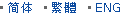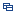| Recent Visit History | Join Audited Suppliers | Help | Region Guide
» » » » Nanjing Product List

121.

## Eyepiece Lens Plano Concave Glass Lens[Nov 20, 2020]

Plano-concave lenses are thicker at the edge than in the center and flat on another surface. Plano concave lens (PCV) has negative focal length. So the lenses are used to bend ...

122.

## Custom Made Optical Glass Bi Convex Lens[Nov 20, 2020]

Double convex lenses have two outward curved surfaces, normally the two surfaces have same radius of curvature, with finely polished. Bi convex lenses have positive focal length, ...

123.

## Glass Lens for Illuminator Condenser[Nov 20, 2020]

Spherical lenses include plano convex lens, plano concave lens, double convex lens, double concave lens, meniscus lens, and achromatic lens. Double convex lenses have two outward ...

124.

## Optical Glass Bk7 Collimating Lens[Nov 20, 2020]

Double convex lenses have two outward curved surfaces, normally the two surfaces have same radius of curvature, with finely polished. Bi convex lenses have positive focal length, ...

125.

## Magnesium Fluoride Glass Bi Convex Lens[Nov 20, 2020]

Double convex lenses have two outward curved surfaces, normally the two surfaces have same radius of curvature, with finely polished. Bi convex lenses have positive focal length, ...

126.

## Manufacturer Plano Concave Lens Double Concave Lens Optical Glass[Nov 20, 2020]

Meniscus lenses are designed to minimize spherical aberration. When used in combination with another lens, meniscus lenses will shorten the focal length and increase the NA of the ...

127.

## Surveying Instrument Optical Glass Plano Convex Lens[Nov 20, 2020]

Double convex lenses have two outward curved surfaces, normally the two surfaces have same radius of curvature, with finely polished. Bi convex lenses have positive focal length, ...

128.

## Optical Glass Bk7 Divergent Lens[Nov 20, 2020]

Double convex lenses have two outward curved surfaces, normally the two surfaces have same radius of curvature, with finely polished. Bi convex lenses have positive focal length, ...

129.

## Optical Glass N-Bk7 Focusing Lens[Nov 20, 2020]

Double convex lenses have two outward curved surfaces, normally the two surfaces have same radius of curvature, with finely polished. Bi convex lenses have positive focal length, ...

130.

## Double Concave Lens Bi Concave Lens Plano Optical Glass[Nov 20, 2020]

N-BK7 bi-concave lenses have equal radius of curvature on both surfaces. Bk7 bi-concave lenses are often used to expand light beams or to increase focal lengths in optical ...

131.

## Optical Glass Bk7 Convergent Lens[Nov 20, 2020]

Double convex lenses have two outward curved surfaces, normally the two surfaces have same radius of curvature, with finely polished. Bi convex lenses have positive focal length, ...

132.

## Factory Offer Glass Bi Convex Lens Custom Made[Nov 20, 2020]

Double convex lenses have two outward curved surfaces, normally the two surfaces have same radius of curvature, with finely polished. Bi convex lenses have positive focal length, ...

133.

## Supplier Optical Glass Bk7 Jgs1 Bi Convex Lens[Nov 20, 2020]

Double convex lenses have two outward curved surfaces, normally the two surfaces have same radius of curvature, with finely polished. Bi convex lenses have positive focal length, ...

134.

## Focusing Glass Lens Bi Convex[Nov 20, 2020]

Bi Convex lens has a positive focal length, and with two outward surfaces. The two surfaces are with same radius of curvature, thereby minimizing spherical aberration. Some ...

135.

## Sapphire Glass Double Convex Lens[Nov 20, 2020]

Double convex lenses comprise two convex spherical surfaces, generally with the same radius of curvature. Bi convex lenses are used as magnifiers, condensing lenses and ...

Hot Region Index: ABCDEFGHIJKLMNPQRSTUWXYZ